A simple explanation to filters, stride and padding in convolution neural networks

We know that CNN use filters to extract feature from the image. This filter moves across the image starting from top left and moving towards right. Once it reaches at the horizontal end of the image, it moves one step vertically downwards and then start moving from left to right. It repeats this process until it reaches at the lower right corner of the image.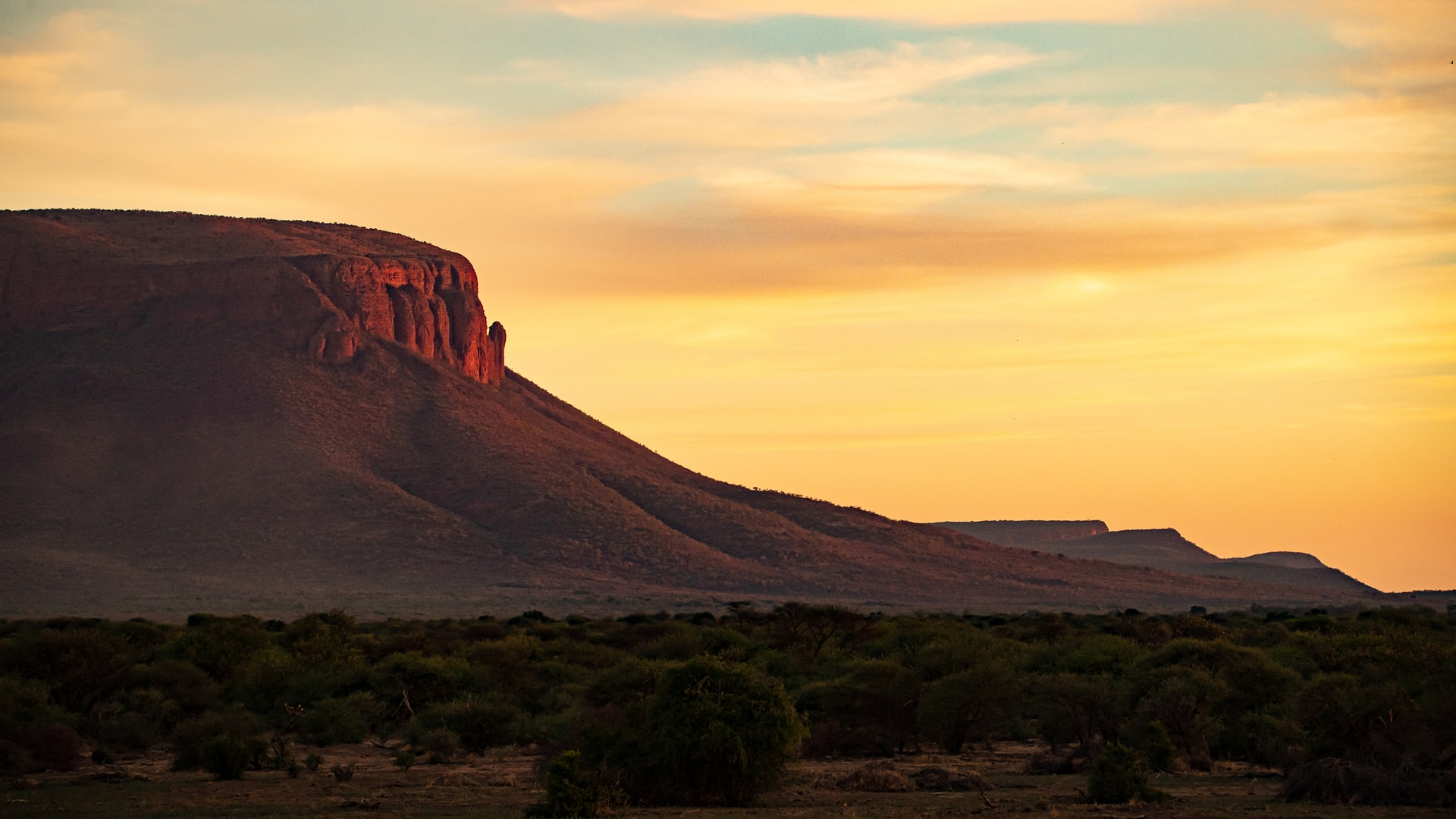Photo by Birger Strahl

Now lets see how these filters extract the features from the image, but before that lets understand what filters actually are?

What are Filters?

Filters are nothing but a matrix of specific number which are when multiplied by an image gives a particular feature map. For example, in the below image when multiplied by a “Right sobel” filter gives an output feature map with vertical lines detected in it.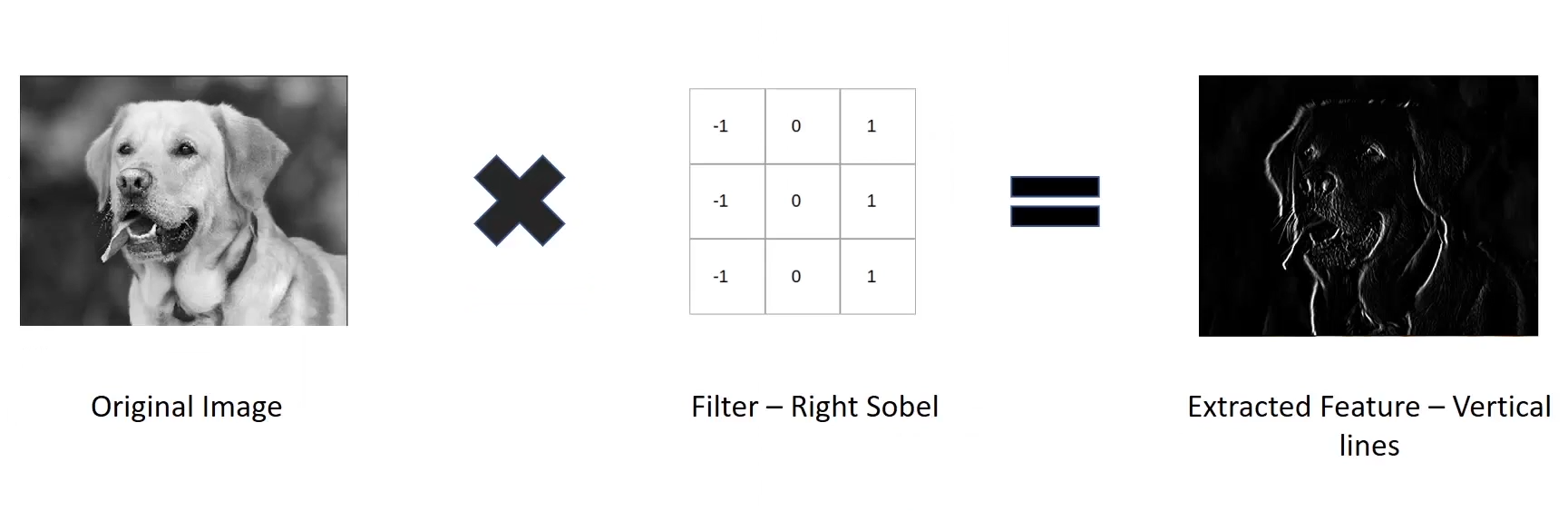Right sobel filter on a 2d image

Different filters have different specific values to detect different features.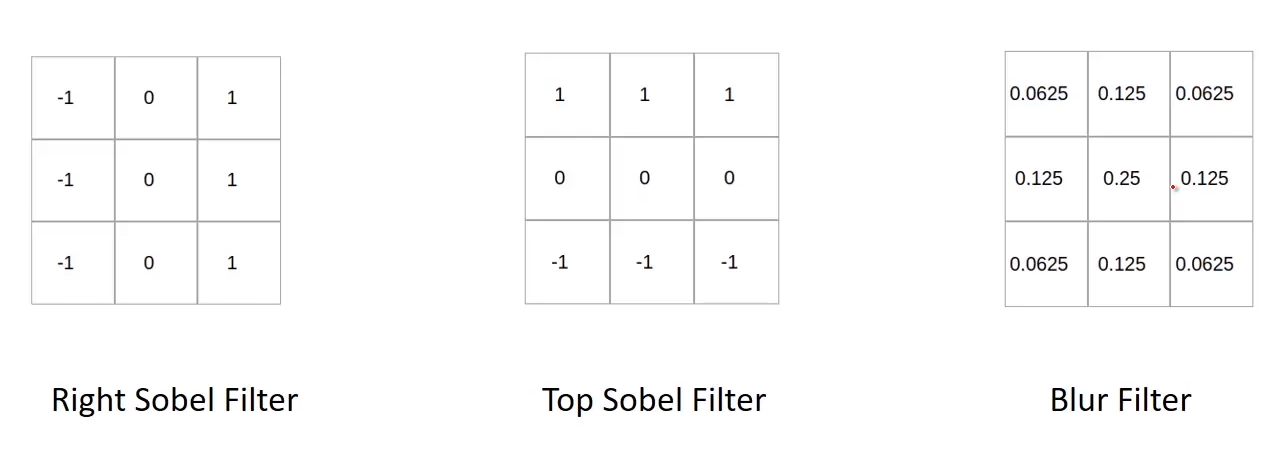Different filter and their values

Now imagine the below image as a image matrix and we pass a right sobel filter to the image. So now the way multiplication works is the filter moves over the image similar to the way we write on a paper. We move left to right move to the next line and continue doing that till we fill up the paper.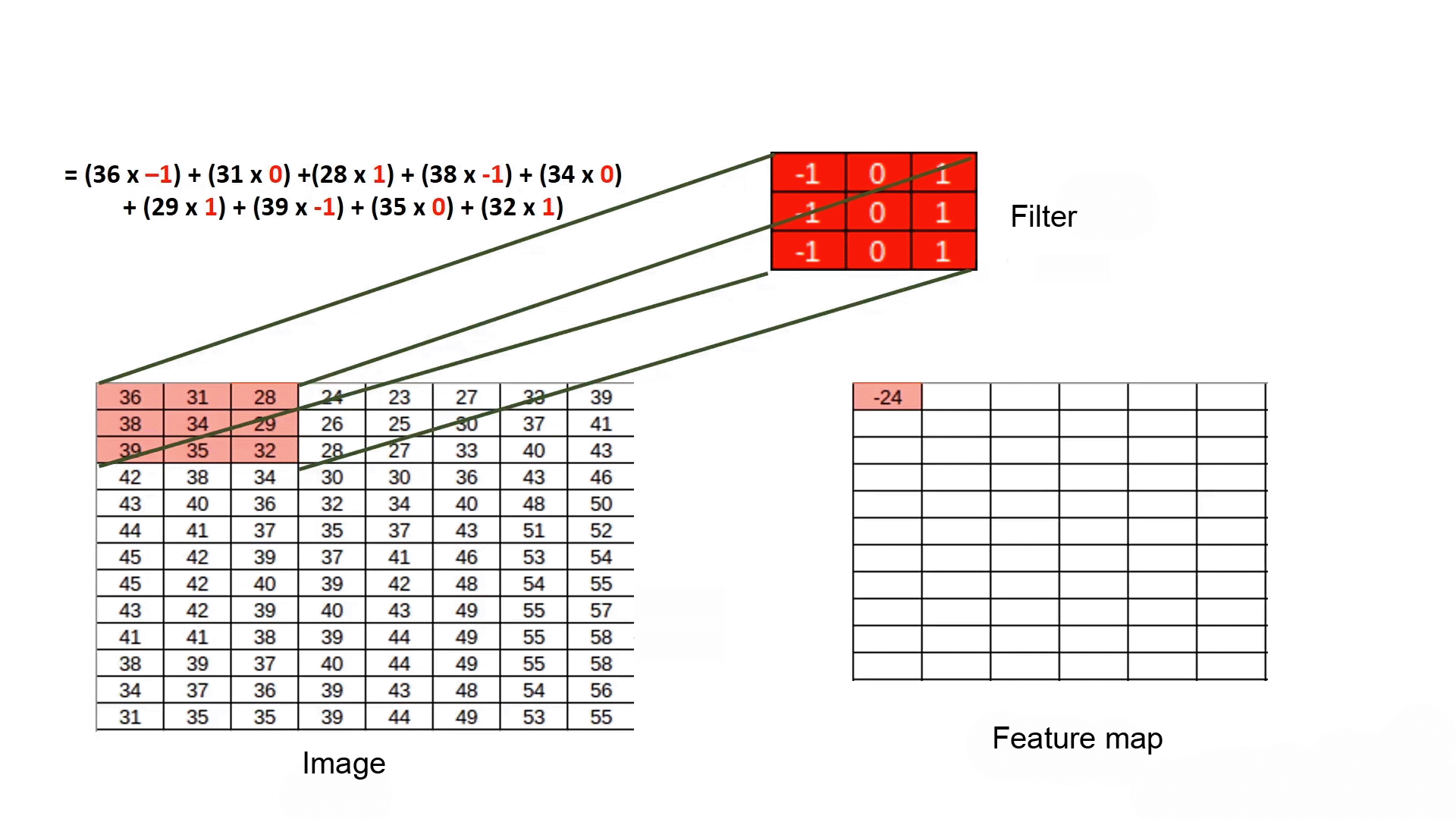Filter working demo

When filter is on the first patch CNN performs element wise multiplication of source image value and filter and then sums it up. This value becomes the value of feature map. Once the CNN is done with calculating the value for the particular patch, the filter moves to the next patch horizontally and repeats this process till the end of the image.

Stride

The number of pixels that the filter moves while moving one step from left to right and top to bottom is know as stride. Below is the example of convolution operation with a filter having stride one.Convolution operation with stride 1

In the above animation we can see that after applying filter the dimension is reduced. To maintain the dimension of output same as the input we use padding.

Suppose you have and image of size 32X32X3 and we pass convolution filter of size 5X5X3. So the output shape will we 28X28X3.

Now again if we apply this filter the shape will be 24X24X3 and if we apply filter once more the shape will be 20X20X3. You can look at the below image for better understanding.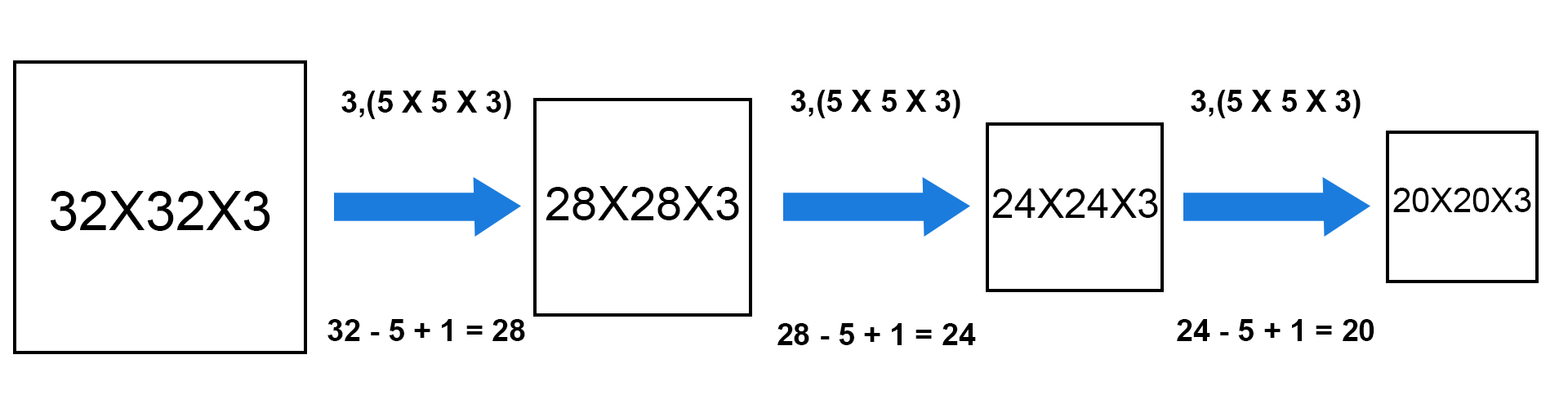Dimensionality reduction due to filters

So, we can see that as we increase the number of filters there is a drastic reduction the size of the output. Image if we have to build a deep neural network with 50 convolution layers, then there will be nothing left in the image to work with.  So, this is the first problem we face while working with the CNNs.

Now again look at the convolution operation with stride 1. The pixels at the corners are used only once to calculate the feature map value. This is the loss of the information.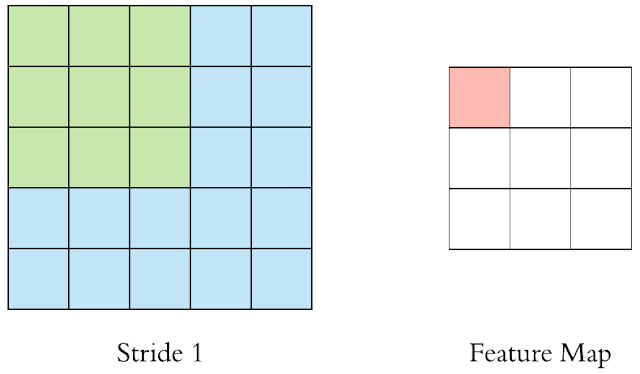Convolution operation with stride 1

Again, consider the below convolution operation with stride 2. In this case the filter never covers the pixels at the right edge of the image and we lose a lot of information.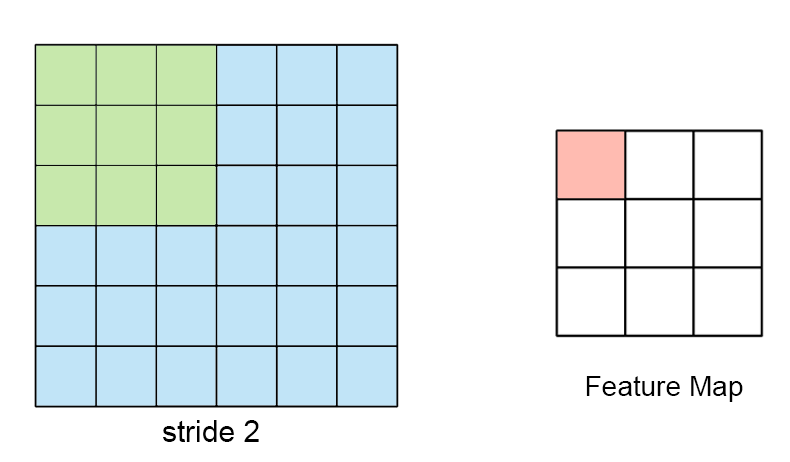Convolution operation with stride 2

To tackle the problem of reduction in dimension and loss of information, we use padding.

In padding we add layers of zeroes around the input image matrix and This layer of zeros is known as padding.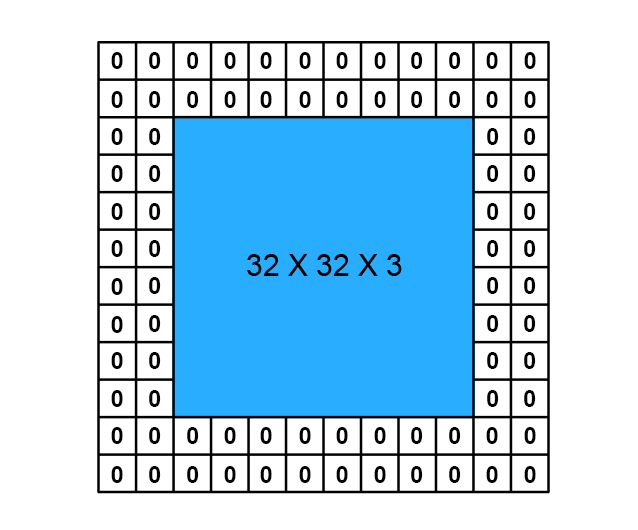Image with padding 2

So, lets assume a 32X32X3 image and we have added a padding of 2 around this image. So now the size of the input image has changed to 36X36X3 and now if we apply a filter of 5X5X3 the output size will be 32X32X3. This way we maintain the size of the output as input.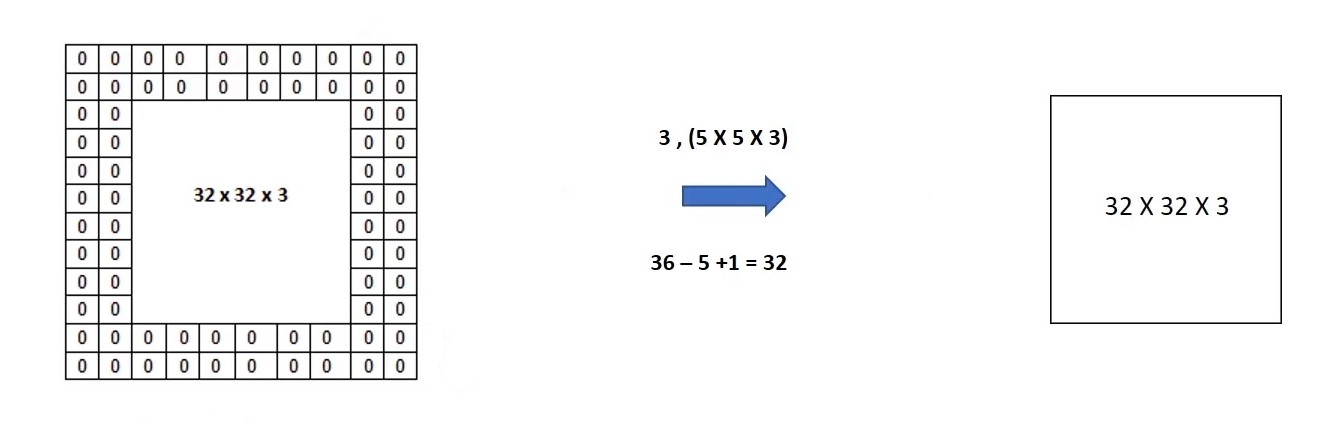Padding working

Along with preserving the dimension of the image the pixels at the corners and edges of the image is also covered by filter equivalently as the other pixels in the image thus no loss of information around the corners and edges of the image.
There are two padding strategies used in CNN.
• Valid: when 'padding = valid', this means that no padding will be applied to the image or there will be no zeros added to the image.
• Same: When "padding = same" this means that padding will be applied to the image or zeros will be added to the image.
One thing to note here is that the padding will keep the output dimension same as input only if stride is 1. If stride is other then 1 then output dimension will be reduced.

So, this is it about CNN filter, stride and padding. I hope you get a good understanding of these terms and how they work? Learn about pooling operation in this article or learn how mean average precision is calculated in object detection with python code.

Understanding mean Average Precision for Object Detection (with Python Code)

Photo by  Avel Chuklanov  on  Unsplash If you ever worked on object detection problem where you need to predict the bounding box coordinates of the objects, you may have come across the term mAP (mean average precision). mAP is a metric used for evaluating object detectors. As the name suggest it is the average of the AP. To understand mAP , first we need to understand what is precision, recall and IoU(Intersection over union). Almost everyone is familiar with first two terms, in case you don’t know these terms I am here to help you. Precision and Recall Precision: It tells us how accurate is our predictions or proportion of data points that our model says relevant are actually relevant. Formula for precision Recall: It is ability of a model to find all the data points of inte